# Lecture 7 - Turing machine

Date Pre-lecture slides Post-lecture scribbles Lecture recording
September 14 2023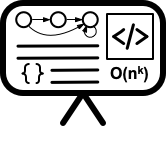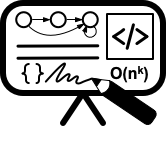## Notes

#### Definition

• Context-sensitive language is a higher level language when compared to Context-Free Language.
• Context-sensitive language is a language that can be generated by some Context Sensitive Grammar and is a set of all languages that can be accepted by a Linear bounded Non-deterministic Turing machine.

#### Definition

A CSG is a quadruple G = (V, T, P, S)

• V = Finite set of non-terminal symbols
• T = Finite set of terminal symbols (alphabet)
• P = Finite set of production rules
• Each production rule is represented as $\alpha$ → $\beta$
• Where $\alpha$ and $\beta$ are strings in $(V \cup T)^*$
• S = S $\in$ V is a start symbol
##### Example

The language L = { $a^{n} b^{n} c^{n}$ | $n \ge 1$} is a context-sensitive language.

• V = {S,A,B}
• T = {a,b,c}
• P = {S → abc | aAbc , Ab → bA , Ac → Bbcc , bB → Bb , aB → aa | aaA}

#### Turing Machines

##### Definition

A Turing machine is a mathematical model of computation describing an abstract machine that manipulates symbols on a strip of tape according to a table of rules. Despite the model’s simplicity, it is capable of implementing any computer algorithm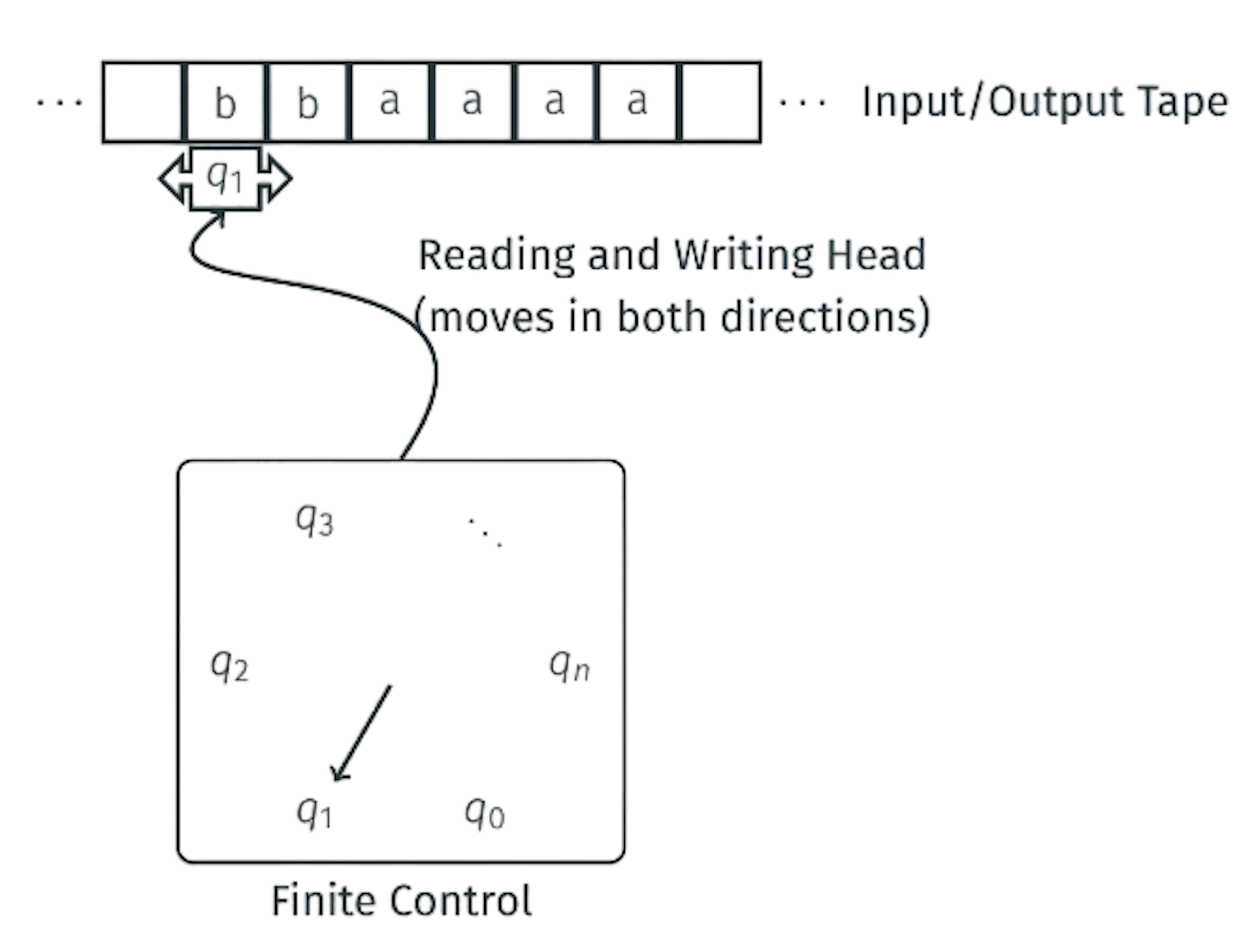• Input written on (infinite) one sided tape.
• Special blank characters
• Finite state control
• Every step: Read character under head, write character out, move the head right or left (or stay).
##### Formal definition

A Turing machine is a 7-tuple(Q, $\Sigma ,\Gamma ,\delta , q_{0}, q_{acc}, q_{rej}$)

• Q : Finite set of states
• $\Sigma$ : Finite input alphabet
• $\Gamma$ : Finite tape alphabet
• $\delta$ : Q x $\Gamma → Q$ x $\Gamma$ x {L, R, S} : Transition function.
• $q_{0}$ $\in$ Q is the initial state
• $q_{acc} \in$ Q is the accepting/final state
• $q_{rej} \in$ Q is the rejecting state
• |_| or ? : Special blank symbol on the tape
##### Transition Function

$\delta$ : Q x $\Gamma → Q$ x $\Gamma$ x {L, R, S}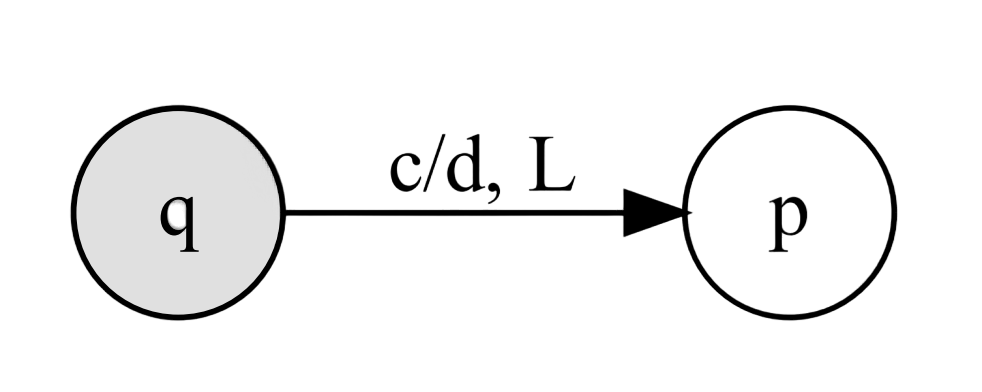Can also be written as c → d, L

$\delta$ : (q, c) = (p, d, L)

• q : Current state.
• c : Character under tape head
• p : New state
• d : Character to write under tape head
• L : Move tape head left
##### Languages defined by a Turing Machine
• A language L is recursively enumerable if L is the set of strings accepted by some Turing Machine. L = {L(M) | M some Turing machine}
• A language L is recursive (decidable) if L is the set of strings accepted by some Turing Machine that halts on every input. L = {L(M) | M some Turing machine that halts on all inputs}.

#### Linear Bounded Automata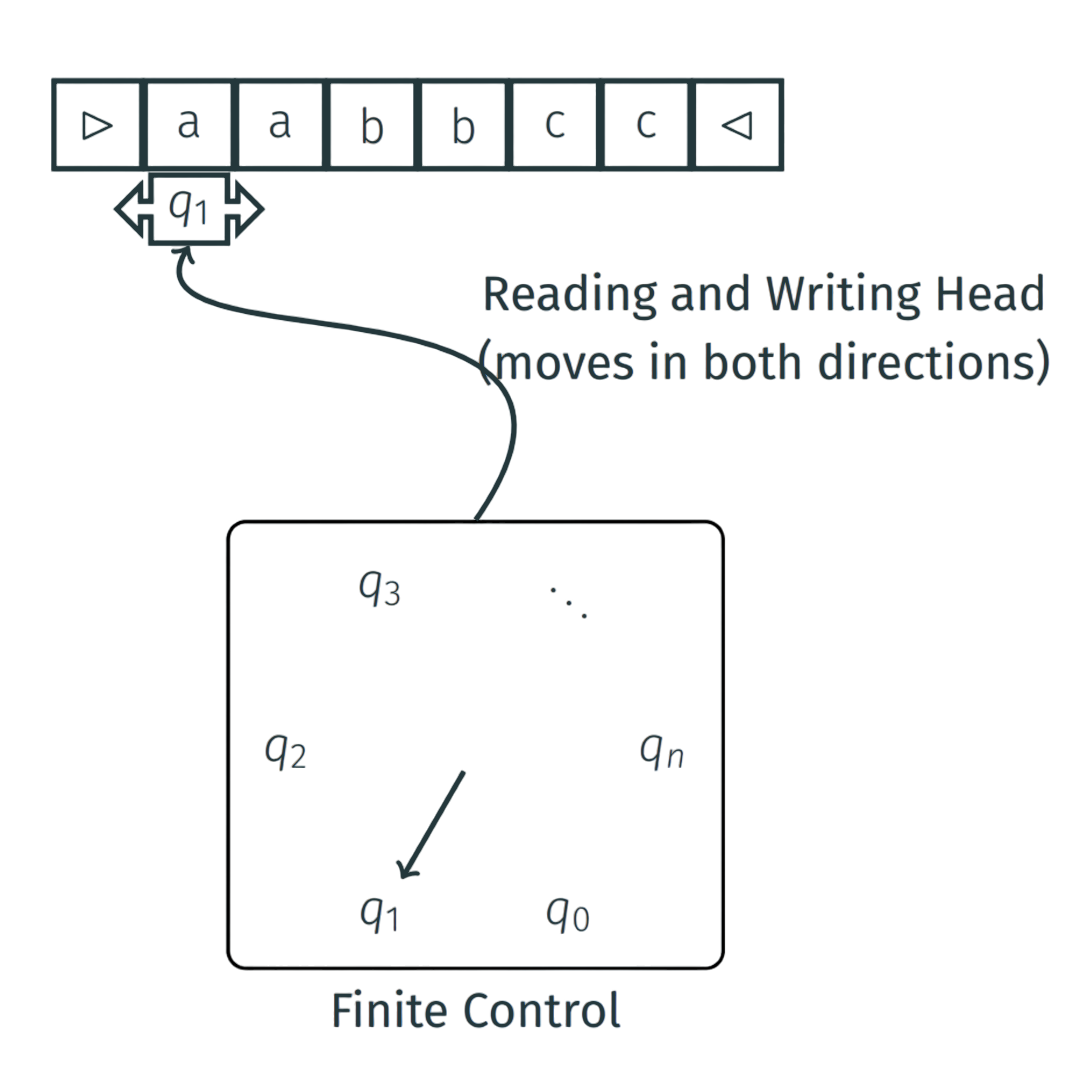• (Nondeterministic) Linear bounded automata can recognize all context sensitive languages.
• Machine can non-deterministically apply all production rule to input in reverse and see if we end up with the start token.